Solving for Boundary Conditions for Second-Order Homogenous ODEs with Constant Coefficients

Second order ODEs require that two conditions  to generate a particular solution.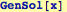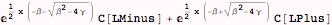Example of y(0) = 0 and y(L)=1: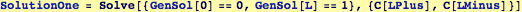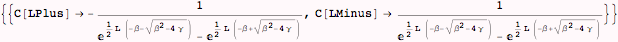Write the resulting solution: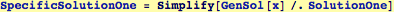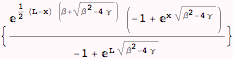Second example:  y(0) = 1 and y'(0)=0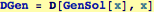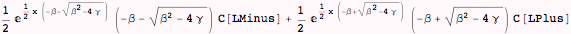Now the constants CPlus and CMinus are found by solving: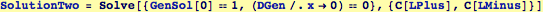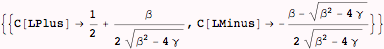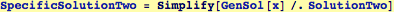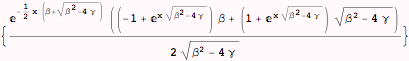Created by Wolfram Mathematica 6.0  (16 November 2007)